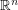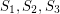# projective plane

## The Double Cap Conjecture ★★

Author(s): Kalai

Conjecture   The largest measure of a Lebesgue measurable subset of the unit sphere ofcontaining no pair of orthogonal vectors is attained by two open caps of geodesic radiusaround the north and south poles.

## Partitioning the Projective Plane ★★

Author(s): Noel

Throughout this post, by projective plane we mean the set of all lines through the origin in.

Definition   Say that a subsetof the projective plane is octahedral if all lines inpass through the closure of two opposite faces of a regular octahedron centered at the origin.
Definition   Say that a subsetof the projective plane is weakly octahedral if every setsuch thatis octahedral.
Conjecture   Suppose that the projective plane can be partitioned into four sets, sayandsuch that each setis weakly octahedral. Then eachis octahedral.

Keywords: Partitioning; projective plane